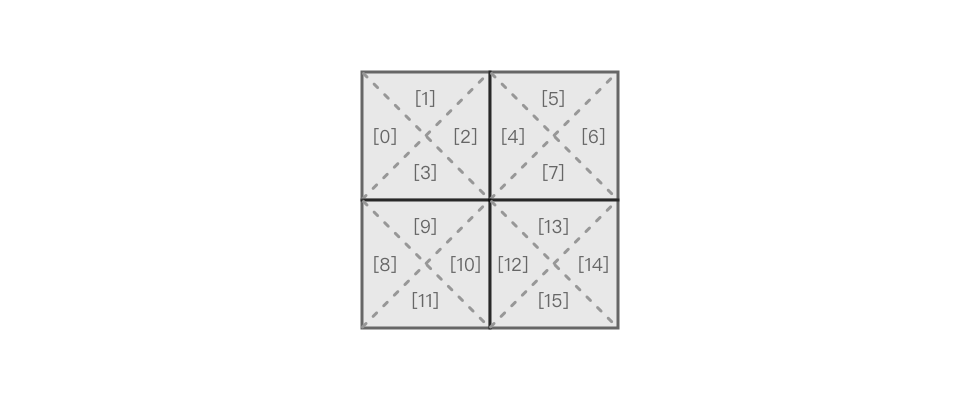0959. 由斜杠划分区域

# 0959. 由斜杠划分区域#

• 标签：深度优先搜索、广度优先搜索、并查集、图
• 难度：中等

## 解题思路 #• 如果是单元格内：
• 如果是空格：合并 indexindex + 1index + 2index + 3
• 如果是 /：合并 indexindex + 1，合并 index + 2index + 3
• 如果是 \\：合并 indexindex + 3，合并 index + 1index + 2
• 如果是单元格间，则向下向右进行合并：
• 向下：合并 index + 3index + 4 * size + 1
• 向右：合并 index + 2index + 4

## 代码 #

  1 2 3 4 5 6 7 8 9 10 11 12 13 14 15 16 17 18 19 20 21 22 23 24 25 26 27 28 29 30 31 32 33 34 35 36 37 38 39 40 41 42 43 44 45 46 47 48 49  class UnionFind: def __init__(self, n): self.parent = [i for i in range(n)] self.count = n def find(self, x): while x != self.parent[x]: self.parent[x] = self.parent[self.parent[x]] x = self.parent[x] return x def union(self, x, y): root_x = self.find(x) root_y = self.find(y) if root_x == root_y: return self.parent[root_x] = root_y self.count -= 1 def is_connected(self, x, y): return self.find(x) == self.find(y) class Solution: def regionsBySlashes(self, grid: List[str]) -> int: size = len(grid) m = 4 * size * size union_find = UnionFind(m) for i in range(size): for j in range(size): index = 4 * (i * size + j) ch = grid[i][j] if ch == '/': union_find.union(index, index + 1) union_find.union(index + 2, index + 3) elif ch == '\\': union_find.union(index, index + 3) union_find.union(index + 1, index + 2) else: union_find.union(index, index + 1) union_find.union(index + 1, index + 2) union_find.union(index + 2, index + 3) if j + 1 < size: union_find.union(index + 2, index + 4) if i + 1 < size: union_find.union(index + 3, index + 4 * size + 1) return union_find.count